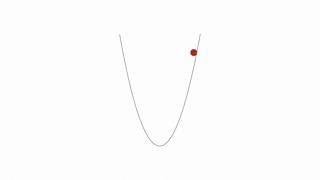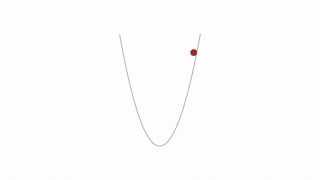# A quick and intuitive explanation of gradient descent

This short writeup is from Udacity’s Deep Learning Nanodegree.

### Journey to the Bottom of the Valley

Here I’ll give you a little refresher on gradient descent so we can start training our network with MiniFlow. Remember that our goal is to make our network output as close as possible to the target values by minimizing the cost. You can envision the cost as a hill or mountain and we want to get to the bottom.

Imagine your model parameters are represented by a ball sitting on a hill. Intuitively, we want to push the ball downhill. And that makes sense, but when we’re talking about our cost function, how do we know which way is downhill?

Luckily, the gradient provides this exact information.

Technically, the gradient actually points uphill, in the direction of steepest ascent. But if we put a `-` sign in front of this value, we get the direction of steepest descent, which is what we want.

You’ll learn more about the gradient in a moment, but, for now, just think of it as a vector of numbers. Each number represents the amount by which we should adjust a corresponding weight or bias in the neural network. Adjusting all of the weights and biases by the gradient values reduces the cost (or error) of the network.

Got all that?

Great! Now we know where to push the ball. The next thing to consider is how much force should be applied to the push. This is known as the learning rate, which is an apt name since this value determines how quickly or slowly the neural network learns.

You might be tempted to set a really big learning rate, so the network learns really fast, right?

Be careful! If the value is too large you could overshoot the target and eventually diverge. Yikes!Convergence. This is the ideal behavior.Divergence. This can happen when the learning rate is too large.

So what is a good learning rate, then?

This is more of a guessing game than anything else but empirically values in the range 0.1 to 0.0001 work well. The range 0.001 to 0.0001 is popular, as 0.1 and 0.01 are sometimes too large.

Here’s the formula for gradient descent (pseudocode):

``````x = x - learning_rate * gradient_of_x
``````

`x` is a parameter used by the neural network (i.e. a single weight or bias).

We multiply `gradient_of_x` (the uphill direction) by `learning_rate` (the force of the push) and then subtract that from `x` to make the push go downhill.

# The Code

Hopefully, you got a basic understanding of gradient descent, a key concept in Machine Learning.

Also published on Medium.